Smartick is an online platform for children to master math in only 15 minutes a day

# I want to learn about: Number & Operations – Fractions

Content about fractions in elementary mathematics. Exercises, tutorials, problems, and teaching resources for fractions in elementary. Here you will find posts about fractions to make learning mathematics easier and more fun. ido generado sobre fracciones de matemáticas de primaria.

Jun21

## Singapore Math Bar Model for Multiplying Fractions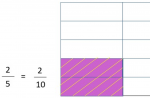In today’s post, we’re going to talk about multiplying fractions using the Singapore Math Bar Model. Let’s take a look at an example: Amy drew a rectangle and colored in 2/5 of the parts purple and after, she striped 1/2 of them yellow. We can calculate the answer by observing the described section between the […]

May22

## Learn and Practice How to Find Sums of Fractions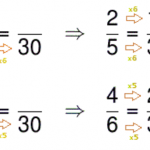Hello! Welcome back to the Smartick blog. In this post, we will look at how to get the sums of fractions. You will see how easy it is for you. To get the sums of fractions, it is necessary that each fraction has the same denominator. Sums of fractions with the same denominator: In this […]

May17

## Singapore Bars Applied to the Addition of Fractions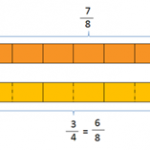In today’s post, we will learn how to apply Singapore Bars to the addition of fractions. You will see how much easier it is working with bars. If you have not heard about the Singapore method and want to know what it is based on, you can look at our blogs on Singapore bars applied […]

May12

## Fractions and Rational Numbers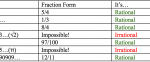We’ve seen a lot of posts on fractions, for example: Adding Fractions, Subtracting Fractions, Dividing Fractions, and Fraction Equivalents… but what exactly are fractions? What kind of number are they? Today, we are going to look at fractions and rational numbers. All fractions are rational numbers. And, what are rational numbers?  They are numbers that […]

May05

## What do people mean by “a Quarter”?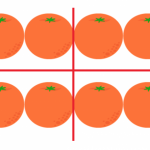Have you ever been told or have you ever read, “It was a quarter of the class,” or “It took me a quarter of what I thought,” or “I have an attendance rate of 25%.”  What do we mean by “quarter?” Today we will discuss a quarter: What it is and what we use it […]

May01

## Learn New Methods for Dividing Fractions

Do you remember how to divide fractions? Today we will look at a new method for dividing fractions.  On this blog, we have seen two methods for dividing fractions: cross multiplication and inversion of fractions and multiplication. Click here to review the methods of dividing fractions: Cross multiply and inversion. To complete any operation with […]

Mar13

## Types of Fractions: Proper, Improper, Fractions Equal to One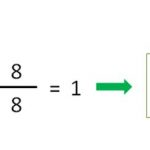Today we will look at the classification of fractions: What types of fractions are there? We can classify them according to the relationship between the numerator and denominator. Proper Fraction Those representing a number less than 1. And how are these fractions identified? All fractions that represent numbers less than 1 are characterized by having […]

Mar09

## Learn about Equivalent Fractions and Practice!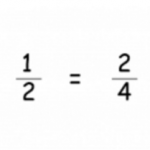What are equivalent fractions? Equivalent fractions are fractions that represent the same quantity, even though the numerator and denominator are different. For example, we have two identical cakes. From one cake, we eat half a piece and from the other, we eat 2 quarters of a piece, which cake has more left? Exactly! Both cakes […]